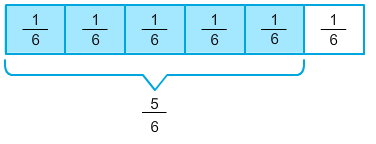# Decompose Fractions

Rate 0 stars
Quiz size:
Message preview:
Someone you know has shared Decompose Fractions quiz with you:

To play this quiz, click on the link below:

https://www.turtlediary.com/quiz/decompose-fractions.html

Hope you have a good experience with this site and recommend to your friends too.

Login to rate activities and track progress.
Login to rate activities and track progress.

To decompose a given fraction into a sum of two, three, or four fractions, we first decompose it into unit fractions.

For example:

Let's decompose the fraction
5 6
into a sum of three fractions.

In order to do so, first let's decompose the given fraction into unit fractions.This model represents a whole divided into 6 equal parts. Here, each part represents the unit fraction
1 6
.The 5 shaded parts represent the fraction
5 6
.Now, write
5 6
as the sum of unit fractions.
 5 6
=
 1 6
+
 1 6
+
 1 6
+
 1 6
+
 1 6

The sum can also be written as:

 5 6
=
 1 6
+
 1 6
+
 1 6
+
 1 6
+
 1 6
=
 1 6
+
 2 6
+
 2 6

So,
5 6
can be written as a sum of three fractions as
1 6
+
2 6
+
2 6
.

ds

A B C D E F G H I J K L M N O P Q R S T U V W X Y Z